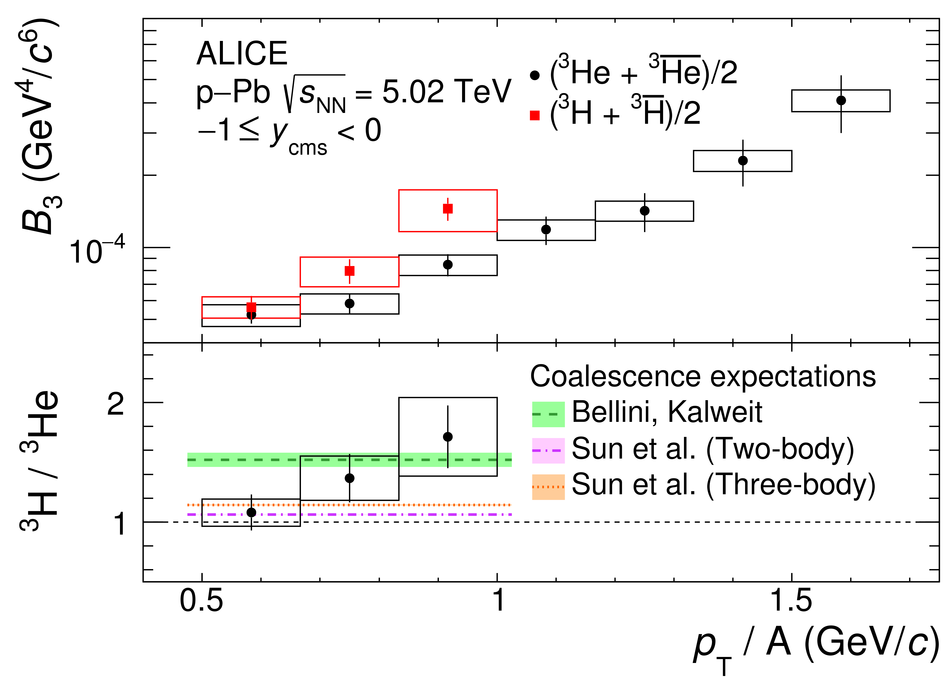# Figure 8

 Top: The coalescence parameter $B_3$ calculated using the average of $\mathrm{INEL}>0$ $^3$He and $^3\overline{\mathrm{He}}$ yields is shown together with the corresponding result for the average of the $^3$H and $^3\overline{\mathrm{H}}$ yields Bottom: The $^3$H/$^3$He yield ratio is shown together with the expectation values from three coalescence approaches . The uncertainties of the coalescence calculations, which are due to the theoretical uncertainties on the emission source radius, are denoted as shaded bands Statistical and systematic uncertainties are indicated by vertical bars and boxes, respectively